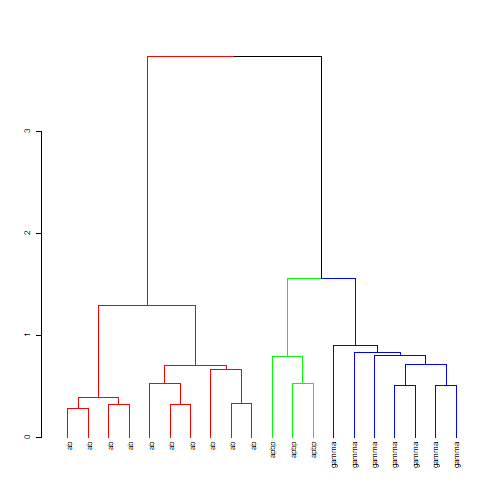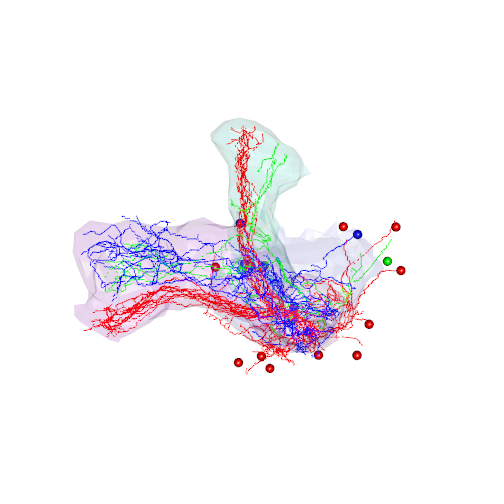# Startup

Install the package if required

``````install.packages('nat.nblast', dependencies = TRUE)
``````

Load the package and set up for knitr / 3D snapshot figures

``````library(nat.nblast)
rgl::setupKnitr()
``````

# Clustering with NBLAST scores

## All by all scores

First we create a 20 x 20 NBLAST all by all score matrix for 20 Kenyon cells included with the nat package

``````library(nat)
kcscores <- nblast_allbyall(kcs20)
``````

## Clustering

Hierarchically cluster the Kenyon scores and divide the clustering into 3 groups

``````hckcs <- nhclust(scoremat=kcscores)
``````
``````## The "ward" method has been renamed to "ward.D"; note new "ward.D2"
``````
``````library(dendroextras)
dkcs <- colour_clusters(hckcs, k=3)
``````

Plot a dendrogram of the clustering, with leaves labelled by true neuron type

``````labels(dkcs) <- with(kcs20[labels(dkcs)], type)
par(cex=.7) # so labels are legible
plot(dkcs)
``````## 3D plot

You can create interactive 3D plots using the rgl package where different subgroups of neurons are coloured according to the calculated clustering.

``````plot3d(hckcs, k=3, db=kcs20, soma=T)
par3d(userMatrix=diag(c(1,-1,-1,1), 4))
plot3d(MBL.surf, alpha=.1)
``````